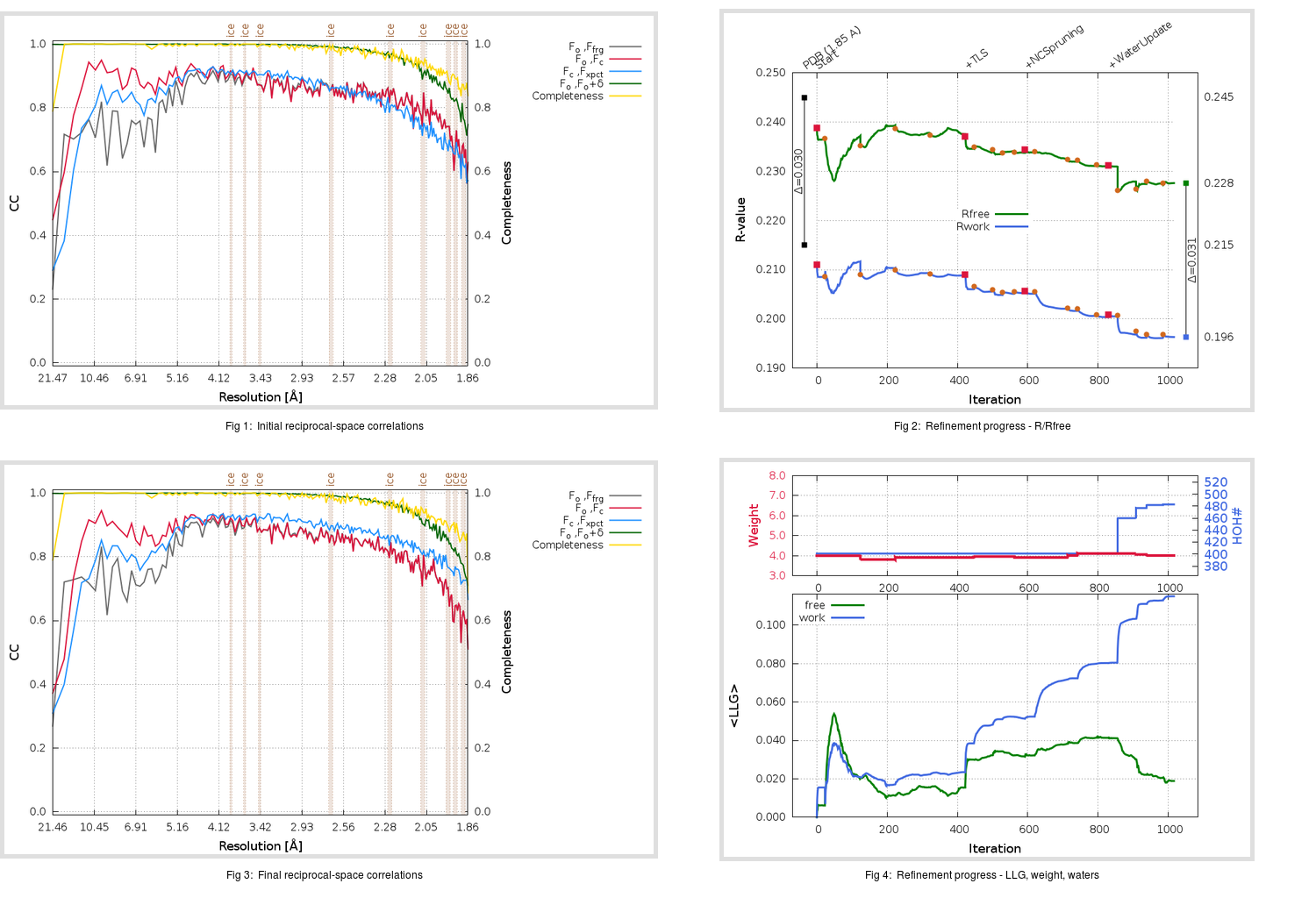Content:

```    Diffraction limits & principal axes of ellipsoid fitted to diffraction cut-off surface:
1.856         0.8980   0.0000   0.4399       0.951 a* + 0.309 c*
1.831         0.0000   1.0000   0.0000       b*
1.823        -0.4399   0.0000   0.8980      -0.329 a* + 0.944 c*
```

## Deposited

` `
 Date deposited Date data collection Resolution R, Rfree 20050809 20041109 1.85 0.2150 0.2450

Molprobity (CCP4 7.0 version) summary:

```Ramachandran outliers =   0.49 %
favored =  97.54 %
Rotamer outliers      =   3.81 %
C-beta deviations     =     0
Clashscore            =  12.98
RMS(bonds)            =   0.0062
RMS(angles)           =   1.42
MolProbity score      =   2.16
Resolution            =   1.85
R-work                =   0.2150
R-free                =   0.2450
```

```Number of waters      =   401

<B> (all atoms) =   28.93 ( sd =    8.62 ) for       5223 non-hydrogen atoms
<B>   (protein) =   28.20 ( sd =    8.35 ) for       4732 non-hydrogen atoms
<B>     (water) =   36.84 ( sd =    7.94 ) for        401 non-hydrogen atoms
<B>    (others) =   32.24 ( sd =    7.15 ) for         90 non-hydrogen atoms

B min/max       (all non-hydrogen atoms) =   15.65 /   65.77
B min/max   (protein non-hydrogen atoms) =   15.65 /   65.77
B min/max     (water non-hydrogen atoms) =   18.67 /   60.80
B min/max     (other non-hydrogen atoms) =   23.00 /   53.28
```

## BUSTER (re-)refinement

` `

Molprobity (CCP4 7.0 version) summary:

```Ramachandran outliers =   0.33 %
favored =  97.87 %
Rotamer outliers      =   4.38 %
C-beta deviations     =     2
Clashscore            =   5.65
RMS(bonds)            =   0.0122
RMS(angles)           =   1.58
MolProbity score      =   1.83
Resolution            =   1.86
R-work                =   0.1963
R-free                =   0.2276
```

```Number of waters      =   483

<B> (all atoms) =   29.76 ( sd =    9.32 ) for       5305 non-hydrogen atoms
<B>   (protein) =   28.72 ( sd =    8.67 ) for       4732 non-hydrogen atoms
<B>     (water) =   39.88 ( sd =    9.93 ) for        483 non-hydrogen atoms
<B>    (others) =   30.20 ( sd =    5.58 ) for         90 non-hydrogen atoms

B min/max       (all non-hydrogen atoms) =   16.86 /   77.41
B min/max   (protein non-hydrogen atoms) =   16.86 /   77.41
B min/max     (water non-hydrogen atoms) =   18.34 /   72.17
B min/max     (other non-hydrogen atoms) =   23.98 /   46.75
```

Refinement progression:Results:

` `
 File Remark 2AMD_aB_refine.01_04_refine.pdb.gz exact refinement commands are in header 2AMD_aB_refine.01_04_refine.mtz.gz including original deposited data and several re-refinement map coefficients 2AMD_aB_refine.01_04_BUSTER_model.cif.gz including any non-standard compound restraints 2AMD_aB_refine.01_04_BUSTER_refln.cif.gz